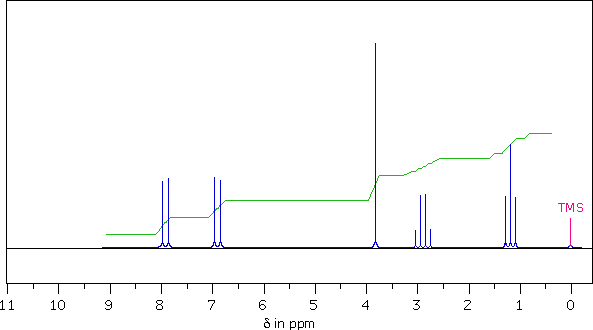Interpreting a NMR Spectrum

The following 1Hnmr spectrum of a C10H12O2 compound was obtained on a 90 MHz spectrometer.
A series of questions are presented below the spectrum. To answer each question enter a number or letter in the designated answer box.
Do not enter punctuation (eg. commas or semicolons) or spaces.1.  Ignoring the TMS signal at δ = 0, how many discrete groups of proton signals are present in this spectrum? ...
2.  What is the multiplicity ( s, d, t, q ) of the highest field signal from this sample? ...
3.  The sample has a singlet at δ = 3.8 ppm. In units of Hertz (Hz) how far is this signal from the TMS signal? ...
4.  What structural feature is suggested by the singlet at δ = 3.8 ppm? ...
A CH3–C=O     B –CH2–     C –O–H     D –O–CH3     E C–CH3     F C=C–H
5.  From multiplet line separations (Js), which of the other signals is coupled to the quartet at δ = 2.9 ppm? ...
A δ = 1.2 ppm     B δ = 3.8 ppm     C δ = 6.9 ppm     D δ = 7.9 ppm

6.  Using the integrator trace and the formula of the sample, assign a whole number ratio to the sample signals as follows:
7.9 ppm signal ;     6.9 ppm signal ;     3.8 ppm signal ;     2.9 ppm signal ;     1.2 ppm signal

 7.  You should now be able to assign a structure to this C10H12O2 compound. To evaluate your assignment Click Here.

This script written by William Reusch, Dept. of Chemistry, Michigan State University.